# Number series generator challenge solutions – Part 3

SentryOne eBooks

In these books, you will find useful, hand-picked articles that will help give insight into some of your most vexing performance problems. These articles were written by several of the SQL Server industry’s leading experts, including Paul White, Paul Randal, Jonathan Kehayias, Erin Stellato, Glenn Berry, Aaron Bertrand, and Joe Sack.

Featured AuthorItzik is a T-SQL trainer, a co-founder of SolidQ, and blogs about T-SQL fundamentals and query tuning.

Itzik’s Posts

This is the third part in a series about solutions to the number series generator challenge. In Part 1 I covered solutions that generate the rows on the fly. In Part 2 I covered solutions that query a physical base table that you prepopulate with rows. This month I’m going to focus on a fascinating technique that can be used to handle our challenge, but that also has interesting applications well beyond it. I’m not aware of an official name for the technique, but it is somewhat similar in concept to horizontal partition elimination, so I’ll refer to it informally as the horizontal unit elimination technique. The technique can have interesting positive performance benefits, but there are also caveats that you need to be aware of, where under certain conditions it can incur a performance penalty.

Thanks again to Alan Burstein, Joe Obbish, Adam Machanic, Christopher Ford, Jeff Moden, Charlie, NoamGr, Kamil Kosno, Dave Mason, John Nelson #2, Ed Wagner, Michael Burbea and Paul White for sharing your ideas and comments.

I’ll do my testing in tempdb, enabling time statistics:

```SET NOCOUNT ON;

USE tempdb;

SET STATISTICS TIME ON;```

## Earlier ideas

The horizontal unit elimination technique can be used as an alternative to the column elimination logic, or vertical unit elimination technique, which I relied on in several of the solutions that I covered earlier. You can read about the fundamentals of column elimination logic with table expressions in Fundamentals of table expressions, Part 3 – Derived tables, optimization considerations under “Column projection and a word on SELECT *.”

The basic idea of the vertical unit elimination technique is that if you have a nested table expression that returns columns x and y, and your outer query references only column x, the query compilation process eliminates y from the initial query tree, and therefore the plan doesn’t need to evaluate it. This has several positive optimization-related implications, such as achieving index coverage with x alone, and if y is a result of a computation, not needing to evaluate y’s underlying expression at all. This idea was at the heart of Alan Burstein’s solution. I also relied on it in several of the other solutions that I covered, such as with the function dbo.GetNumsAlanCharlieItzikBatch (from Part 1), the functions dbo.GetNumsJohn2DaveObbishAlanCharlieItzik and dbo.GetNumsJohn2DaveObbishAlanCharlieItzik2 (from Part 2), and others. As an example, I’ll use dbo.GetNumsAlanCharlieItzikBatch as the baseline solution with the vertical elimination logic.

As a reminder, this solution uses a join with a dummy table that has a columnstore index to get batch processing. Here’s the code to create the dummy table:

```DROP TABLE IF EXISTS dbo.BatchMe;
GO

CREATE TABLE dbo.BatchMe(col1 INT NOT NULL, INDEX idx_cs CLUSTERED COLUMNSTORE);```

And here’s the code with the definition of the dbo.GetNumsAlanCharlieItzikBatch function:

```CREATE OR ALTER FUNCTION dbo.GetNumsAlanCharlieItzikBatch(@low AS BIGINT = 1, @high AS BIGINT)
RETURNS TABLE
AS
RETURN
WITH
L0 AS ( SELECT 1 AS c
FROM (VALUES(1),(1),(1),(1),(1),(1),(1),(1),
(1),(1),(1),(1),(1),(1),(1),(1)) AS D(c) ),
L1 AS ( SELECT 1 AS c FROM L0 AS A CROSS JOIN L0 AS B ),
L2 AS ( SELECT 1 AS c FROM L1 AS A CROSS JOIN L1 AS B ),
L3 AS ( SELECT 1 AS c FROM L2 AS A CROSS JOIN L2 AS B ),
Nums AS ( SELECT ROW_NUMBER() OVER(ORDER BY (SELECT NULL)) AS rownum
FROM L3 )
SELECT TOP(@high - @low + 1)
rownum AS rn,
@high + 1 - rownum AS op,
@low - 1 + rownum AS n
FROM Nums LEFT OUTER JOIN dbo.BatchMe ON 1 = 0
ORDER BY rownum;
GO```

I used the following code to test the function's performance with 100M rows, returning the computed result column n (manipulation of the result of the ROW_NUMBER function), ordered by n:

```DECLARE @n AS BIGINT;

SELECT @n = n FROM dbo.GetNumsAlanCharlieItzikBatch(1, 100000000) ORDER BY n OPTION(MAXDOP 1);```

Here are the time statistics that I got for this test:

CPU time = 9328 ms, elapsed time = 9330 ms.

I used the following code to test the function's performance with 100M rows, returning the column rn (direct, unmanipulated, result of the ROW_NUMBER function), ordered by rn:

```DECLARE @n AS BIGINT;

SELECT @n = rn FROM dbo.GetNumsAlanCharlieItzikBatch(1, 100000000) ORDER BY rn OPTION(MAXDOP 1);```

Here are the time statistics that I got for this test:

CPU time = 7296 ms, elapsed time = 7291 ms.

Let’s review the important ideas that are embedded in this solution.

Relying on column elimination logic, alan came up with the idea to return not just one column with the number series, but three:

• Column rn represents an unmanipulated result of the ROW_NUMBER function, which starts with 1. It is cheap to compute. It is order preserving both when you provide constants and when you provide nonconstants (variables, columns) as inputs to the function. This means that when your outer query uses ORDER BY rn, you don’t get a Sort operator in the plan.
• Column n represents a computation based on @low, a constant, and rownum (result of the ROW_NUMBER function). It is order preserving with respect to rownum when you provide constants as inputs to the function. That’s thanks to Charlie’s insight regarding constant folding (see Part 1 for details). However, it is not order preserving when you provide nonconstants as inputs, since you don’t get constant folding. I’ll demonstrate this later in the section about caveats.
• Column op represents n in opposite order. It is a result of a computation and it is not order preserving.

Relying on the column elimination logic, if you need to return a number series starting with 1, you query column rn, which is cheaper than querying n. If you need a number series starting with a value other than 1, you query n and pay the extra cost. If you need the result ordered by the number column, with constants as inputs you can use either ORDER BY rn or ORDER BY n. But with nonconstants as inputs, you want to make sure to use ORDER BY rn. It might be a good idea to just always stick to using ORDER BY rn when needing the result ordered to be on the safe side.

The horizontal unit elimination idea is similar to the vertical unit elimination idea, only it applies to sets of rows instead of sets of columns. In fact, Joe Obbish relied on this idea in his function dbo.GetNumsObbish (from Part 2), and we will take it a step further. In his solution, Joe unified multiple queries representing disjoint subranges of numbers, using a filter in the WHERE clause of each query to define the applicability of the subrange. When you call the function and pass constant inputs representing the delimiters of your desired range, SQL Server eliminates the inapplicable queries at compile time, so the plan doesn’t even reflect them.

## Horizontal unit elimination, compile time versus run time

Perhaps it would be a good idea to start by demonstrating the concept of horizontal unit elimination in a more general case, and also discuss an important distinction between compile-time and run-time elimination. Then we can discuss how to apply the idea to our number series challenge.

I’ll use three tables called dbo.T1, dbo.T2 and dbo.T3 in my example. Use the following DDL and DML code to create and populate these tables:

```DROP TABLE IF EXISTS dbo.T1, dbo.T2, dbo.T3;
GO

CREATE TABLE dbo.T1(col1 INT); INSERT INTO dbo.T1(col1) VALUES(1);
CREATE TABLE dbo.T2(col1 INT); INSERT INTO dbo.T2(col1) VALUES(2);
CREATE TABLE dbo.T3(col1 INT); INSERT INTO dbo.T3(col1) VALUES(3);```

Suppose that you want to implement an inline TVF called dbo.OneTable that accepts one of the above three table names as input, and returns the data from the requested table. Based on the horizontal unit elimination concept, you could implement the function like so:

```CREATE OR ALTER FUNCTION dbo.OneTable(@WhichTable AS NVARCHAR(257))
RETURNS TABLE
AS
RETURN
SELECT col1 FROM dbo.T1 WHERE @WhichTable = N'dbo.T1'
UNION ALL
SELECT col1 FROM dbo.T2 WHERE @WhichTable = N'dbo.T2'
UNION ALL
SELECT col1 FROM dbo.T3 WHERE @WhichTable = N'dbo.T3';
GO```

Remember that an inline TVF applies parameter embedding. This means that when you pass a constant such as N'dbo.T2' as input, the inlining process substitutes all references to @WhichTable with the constant prior to optimization. The elimination process can then remove the references to T1 and T3 from the initial query tree, and thus query optimization results in a plan that references only T2. Let’s test this idea with the following query:

`SELECT * FROM dbo.OneTable(N'dbo.T2');`

The plan for this query is shown in Figure 1.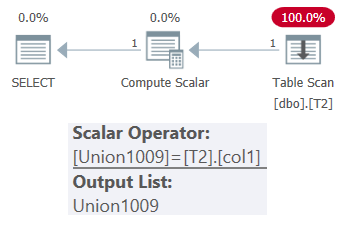Figure 1: Plan for dbo.OneTable with constant input

As you can see, only table T2 appears in the plan.

Things are a bit trickier when you pass a nonconstant as input. This could be the case when using a variable, a procedure parameter, or passing a column via APPLY. The input value is either unknown at compile time, or parameterized plan reuse potential needs to be taken into account.

The optimizer cannot eliminate any of the tables from the plan, but it still has a trick. It can use startup Filter operators above the subtrees that access the tables, and execute only the relevant subtree based on the runtime value of @WhichTable. Use the following code to test this strategy:

```DECLARE @T AS NVARCHAR(257) = N'dbo.T2';

SELECT * FROM dbo.OneTable(@T);```

The plan for this execution is shown in Figure 2: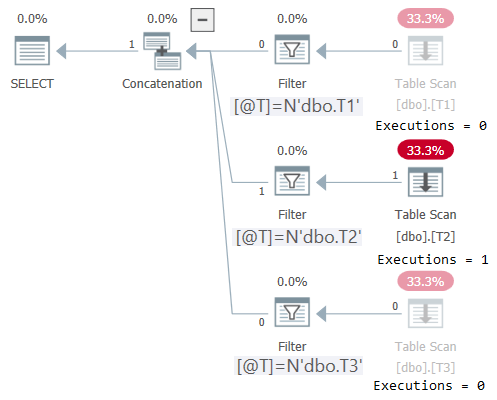Figure 2: Plan for dbo.OneTable with nonconstant input

Plan Explorer makes it wonderfully obvious to see that only the applicable subtree got executed (Executions = 1), and grays out the subtrees that didn’t get executed (Executions = 0). Also, STATISTICS IO shows I/O info only for the table that was accessed:

## Applying horizontal unit elimination logic to the number series challenge

As mentioned, you can apply the horizontal unit elimination concept by modifying any of the earlier solutions that currently use vertical elimination logic. I’ll use the function dbo.GetNumsAlanCharlieItzikBatch as the starting point for my example.

Recall that Joe Obbish used horizontal unit elimination to extract the relevant disjoint subranges of the number series. We will use the concept to horizontally separate the less expensive computation (rn) where @low = 1 from the more expensive computation (n) where @low <> 1.

While we’re at it, we can experiment by adding Jeff Moden’s idea in his fnTally function, where he uses a sentinel row with the value 0 for cases where the range starts with @low = 0.

So we have four horizontal units:

• Sentinel row with 0 where @low = 0, with n = 0
• TOP (@high) rows where @low = 0, with cheap n = rownum, and op = @high – rownum
• TOP (@high) rows where @low = 1, with cheap n = rownum, and op = @high + 1 – rownum
• TOP(@high – @low + 1) rows where @low <> 0 AND @low <> 1, with more expensive n = @low – 1 + rownum, and op = @high + 1 – rownum

This solution combines ides from Alan, Charlie, Joe, Jeff and myself, so we’ll call the batch-mode version of the function dbo.GetNumsAlanCharlieJoeJeffItzikBatch.

First, remember to make sure that you still have the dummy table dbo.BatchMe present to get batch processing in our solution, or use the following code if you don’t:

```DROP TABLE IF EXISTS dbo.BatchMe;
GO

CREATE TABLE dbo.BatchMe(col1 INT NOT NULL, INDEX idx_cs CLUSTERED COLUMNSTORE);```

Here’s the code with the definition of dbo.GetNumsAlanCharlieJoeJeffItzikBatch function:

```CREATE OR ALTER FUNCTION dbo.GetNumsAlanCharlieJoeJeffItzikBatch(@low AS BIGINT = 1, @high AS BIGINT)
RETURNS TABLE
AS
RETURN
WITH
L0 AS ( SELECT 1 AS c
FROM (VALUES(1),(1),(1),(1),(1),(1),(1),(1),
(1),(1),(1),(1),(1),(1),(1),(1)) AS D(c) ),
L1 AS ( SELECT 1 AS c FROM L0 AS A CROSS JOIN L0 AS B ),
L2 AS ( SELECT 1 AS c FROM L1 AS A CROSS JOIN L1 AS B ),
L3 AS ( SELECT 1 AS c FROM L2 AS A CROSS JOIN L2 AS B ),
Nums AS ( SELECT ROW_NUMBER() OVER(ORDER BY (SELECT NULL)) AS rownum
FROM L3 )
SELECT @low AS n, @high AS op WHERE @low = 0 AND @high > @low
UNION ALL
SELECT TOP(@high)
rownum AS n,
@high - rownum AS op
FROM Nums LEFT OUTER JOIN dbo.BatchMe ON 1 = 0
WHERE @low = 0
ORDER BY rownum
UNION ALL
SELECT TOP(@high)
rownum AS n,
@high + 1 - rownum AS op
FROM Nums LEFT OUTER JOIN dbo.BatchMe ON 1 = 0
WHERE @low = 1
ORDER BY rownum
UNION ALL
SELECT TOP(@high - @low + 1)
@low - 1 + rownum AS n,
@high + 1 - rownum AS op
FROM Nums LEFT OUTER JOIN dbo.BatchMe ON 1 = 0
WHERE @low <> 0 AND @low <> 1
ORDER BY rownum;
GO```

Important: The horizontal unit elimination concept is doubtless more complex to implement than the vertical one, so why bother? Because it removes the responsibility to choose the right column from the user. The user only needs to worry about querying a column called n, as opposed to remembering to use rn when the range starts with 1, and n otherwise.

Let’s start by testing the solution with constant inputs 1 and 100,000,000, asking for the result to be ordered:

```DECLARE @n AS BIGINT;

SELECT @n = n FROM dbo.GetNumsAlanCharlieJoeJeffItzikBatch(1, 100000000) ORDER BY n OPTION(MAXDOP 1);```

The plan for this execution is shown in Figure 3.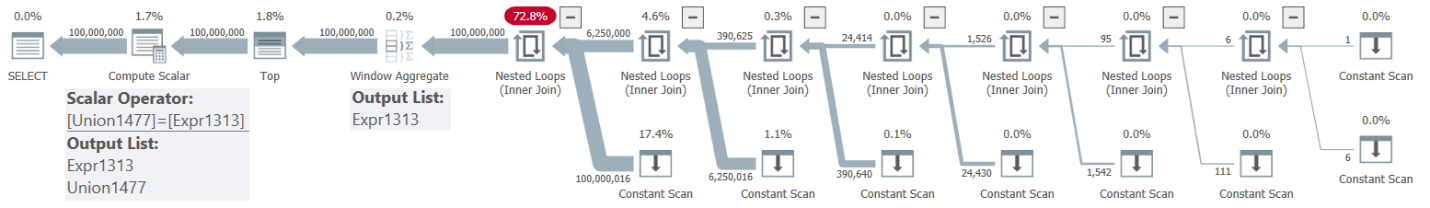Figure 3: Plan for dbo.GetNumsAlanCharlieJoeJeffItzikBatch(1, 100M)

Observe that the only returned column is based on the direct, unmanipulated, ROW_NUMBER expression (Expr1313). Also observe that there’s no need for sorting in the plan.

I got the following time statistics for this execution:

CPU time = 7359 ms, elapsed time = 7354 ms.

The runtime adequately reflect the fact that the plan uses batch mode, the unmanipulated ROW_NUMBER expression, and no sorting.

Next, test the function with the constant range 0 to 99,999,999:

```DECLARE @n AS BIGINT;

SELECT @n = n FROM dbo.GetNumsAlanCharlieJoeJeffItzikBatch(0, 99999999) ORDER BY n OPTION(MAXDOP 1);```

The plan for this execution is shown in Figure 4.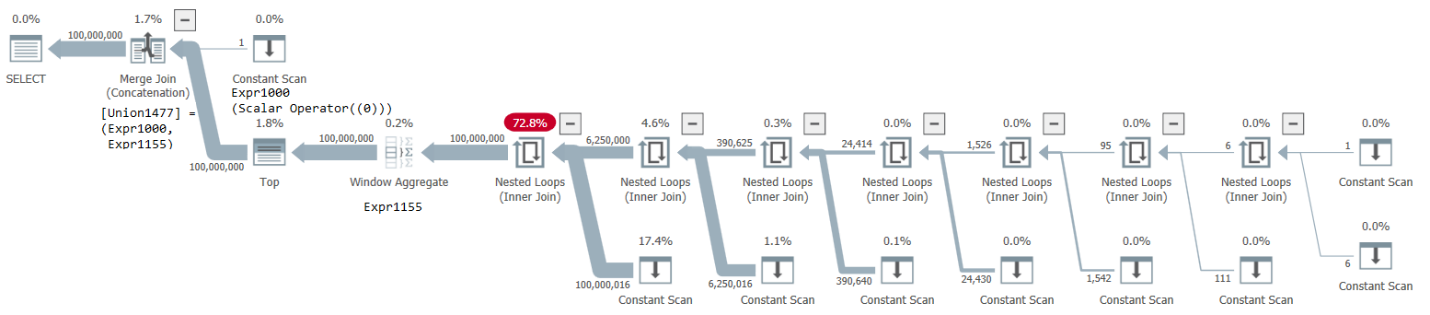Figure 4: Plan for dbo.GetNumsAlanCharlieJoeJeffItzikBatch(0, 99999999)

The plan uses a Merge Join (Concatenation) operator to merge the sentinel row with the value 0 and the rest. Even though the second part is as efficient as before, the merge logic takes a pretty big toll of about 26% on the run time, resulting in the following time stats:

CPU time = 9265 ms, elapsed time = 9298 ms.

Let’s test the function with the constant range 2 to 100,000,001:

```DECLARE @n AS BIGINT;

SELECT @n = n FROM dbo.GetNumsAlanCharlieJoeJeffItzikBatch(2, 100000001) ORDER BY n OPTION(MAXDOP 1);```

The plan for this execution is shown in Figure 5.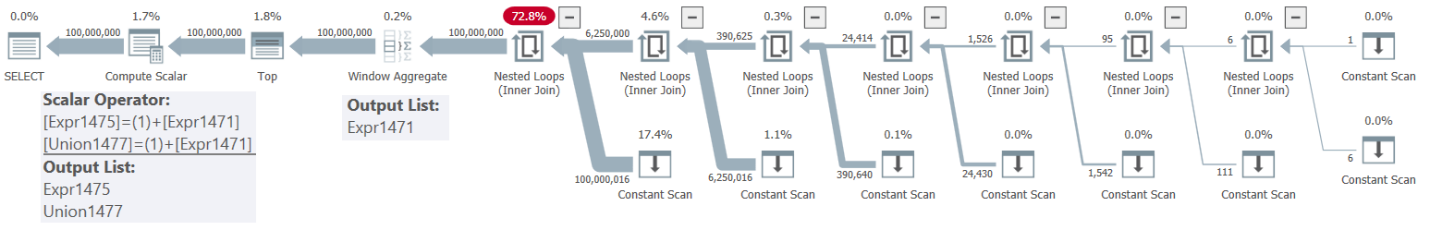Figure 5: Plan for dbo.GetNumsAlanCharlieJoeJeffItzikBatch(2, 100000001)

This time there’s no expensive merge logic since the sentinel row part is irrelevant. However, observe that the returned column is the manipulated expression @low – 1 + rownum, which after parameter embedding/inlining and constant folding became 1 + rownum.

Here are the time statistics that I got for this execution:

CPU Time = 9000 ms, elapsed time = 9015 ms.

As expected, this is not as fast as with a range that starts with 1, but interestingly, faster than with a range that starts with 0.

## Removing the 0 sentinel row

Given that the technique with the sentinel row with the value 0 seems to be slower than applying manipulation to rownum, it makes sense to simply avoid it. This brings us to a simplified horizontal elimination-based solution that mixes the ideas from Alan, Charlie, Joe and myself. I’ll call the function with this solution dbo.GetNumsAlanCharlieJoeItzikBatch. Here’s the function’s definition:

```CREATE OR ALTER FUNCTION dbo.GetNumsAlanCharlieJoeItzikBatch(@low AS BIGINT = 1, @high AS BIGINT)
RETURNS TABLE
AS
RETURN
WITH
L0 AS ( SELECT 1 AS c
FROM (VALUES(1),(1),(1),(1),(1),(1),(1),(1),
(1),(1),(1),(1),(1),(1),(1),(1)) AS D(c) ),
L1 AS ( SELECT 1 AS c FROM L0 AS A CROSS JOIN L0 AS B ),
L2 AS ( SELECT 1 AS c FROM L1 AS A CROSS JOIN L1 AS B ),
L3 AS ( SELECT 1 AS c FROM L2 AS A CROSS JOIN L2 AS B ),
Nums AS ( SELECT ROW_NUMBER() OVER(ORDER BY (SELECT NULL)) AS rownum
FROM L3 )
SELECT TOP(@high)
rownum AS n,
@high + 1 - rownum AS op
FROM Nums LEFT OUTER JOIN dbo.BatchMe ON 1 = 0
WHERE @low = 1
ORDER BY rownum
UNION ALL
SELECT TOP(@high - @low + 1)
@low - 1 + rownum AS n,
@high + 1 - rownum AS op
FROM Nums LEFT OUTER JOIN dbo.BatchMe ON 1 = 0
WHERE @low <> 1
ORDER BY rownum;
GO```

Let’s test it with the range 1 to 100M:

```DECLARE @n AS BIGINT;

SELECT @n = n FROM dbo.GetNumsAlanCharlieJoeItzikBatch(1, 100000000) ORDER BY n OPTION(MAXDOP 1);```

The plan is the same as the one shown earlier in Figure 3, as expected.

Accordingly, I got the following time stats:

CPU time = 7219 ms, elapsed time = 7243 ms.

Test it with the range 0 to 99,999,999:

```DECLARE @n AS BIGINT;

SELECT @n = n FROM dbo.GetNumsAlanCharlieJoeItzikBatch(0, 99999999) ORDER BY n OPTION(MAXDOP 1);```

This time you get the same plan as the one shown earlier in Figure 5—not Figure 4.

Here are the time stats that I got for this execution:

CPU Time = 9313 ms, elapsed time = 9334 ms.

Test it with the range 2 to 100,000,001:

```DECLARE @n AS BIGINT;

SELECT @n = n FROM dbo.GetNumsAlanCharlieJoeItzikBatch(2, 100000001) ORDER BY n OPTION(MAXDOP 1);```

Again you get the same plan as the one shown earlier in Figure 5.

I got the following time statistics for this execution:

CPU Time = 9125 ms, elapsed time = 9148 ms.

## Caveats when using nonconstant inputs

With both the vertical and horizontal unit elimination techniques, things works ideally as long as you pass constants as inputs. However, you do need to be aware of caveats that may result in performance penalties when you pass nonconstant inputs. The vertical unit elimination technique has fewer issues, and the issues that do exists are easier to cope with, so let’s start with it.

Remember that in this article we used the function dbo.GetNumsAlanCharlieItzikBatch as our example that relies on the vertical unit elimination concept. Let’s run a series of tests with nonconstant inputs, such as variables.

As our first test, we’ll return rn and ask for the data ordered by rn:

```DECLARE @mylow AS BIGINT = 1, @myhigh AS BIGINT = 100000000;

DECLARE @n AS BIGINT;

SELECT @n = rn FROM dbo.GetNumsAlanCharlieItzikBatch(@mylow, @myhigh) ORDER BY rn OPTION(MAXDOP 1);```

Remember that rn represents the unmanipulated ROW_NUMBER expression, so the fact that we use nonconstant inputs has no special significance in this case. There’s no need for explicit sorting in the plan.

I got the following time stats for this execution:

CPU Time = 7390 ms, elapsed time = 7386 ms.

These numbers represent the ideal case.

In the next test, order the result rows by n:

```DECLARE @mylow AS BIGINT = 1, @myhigh AS BIGINT = 100000000;

DECLARE @n AS BIGINT;

SELECT @n = n FROM dbo.GetNumsAlanCharlieItzikBatch(@mylow, @myhigh) ORDER BY n OPTION(MAXDOP 1);```

The plan for this execution is shown in Figure 6.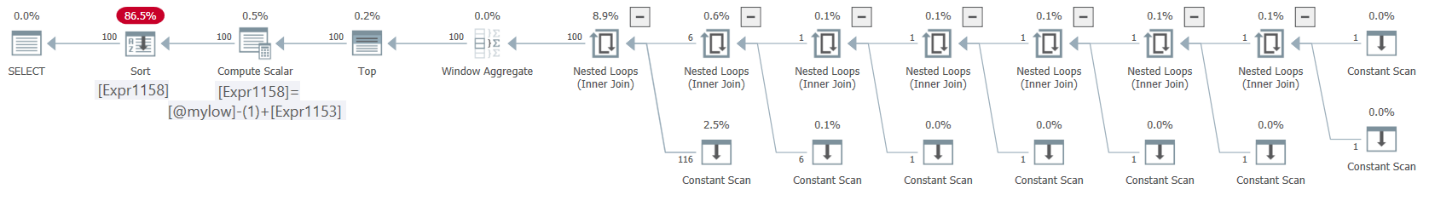Figure 6: Plan for dbo.GetNumsAlanCharlieItzikBatch(@mylow, @myhigh) ordering by n

See the problem? After inlining, @low was substituted with @mylow—not with the value in @mylow, which is 1. Consequently, constant folding didn’t take place, and therefore n is not order preserving with respect to rownum. This resulted in explicit sorting in the plan.

Here are the time statistics that I got for this execution:

CPU Time = 25141 ms, elapsed time = 25628 ms.

Execution time almost tripled compared to when explicit sorting wasn’t needed.

A simple workaround is to use Alan Burstein’s original idea to always order by rn when you need the result ordered, both when returning rn and when returning n, like so:

```DECLARE @mylow AS BIGINT = 1, @myhigh AS BIGINT = 100000000;

DECLARE @n AS BIGINT;

SELECT @n = n FROM dbo.GetNumsAlanCharlieItzikBatch(@mylow, @myhigh) ORDER BY rn OPTION(MAXDOP 1);```

This time there’s no explicit sorting in the plan.

I got the following time statistics for this execution:

CPU Time = 9156 ms, elapsed time = 9184 ms.

The numbers adequately reflect the fact that you are returning the manipulated expression, but incurring no explicit sorting.

With solutions that are based on the horizontal unit elimination technique, such as our dbo.GetNumsAlanCharlieJoeItzikBatch function, the situation is more complicated when using nonconstant inputs.

Let’s first test the function with a very small range of 10 numbers:

```DECLARE @mylow AS BIGINT = 1, @myhigh AS BIGINT = 10;

DECLARE @n AS BIGINT;

SELECT @n = n FROM dbo.GetNumsAlanCharlieJoeItzikBatch(@mylow, @myhigh) ORDER BY n OPTION(MAXDOP 1);```

The plan for this execution is shown in Figure 7.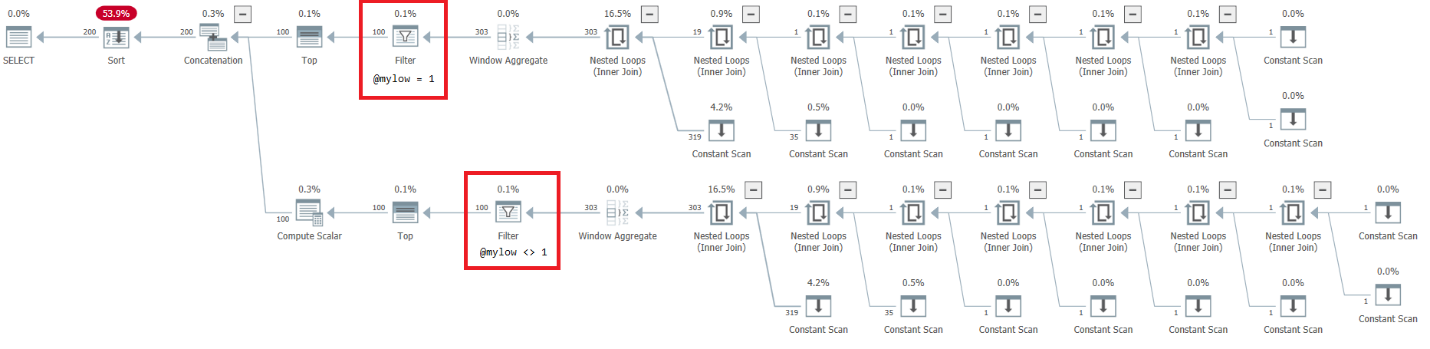Figure 7: Plan for dbo.GetNumsAlanCharlieJoeItzikBatch(@mylow, @myhigh)

There’s a very alarming side to this plan. Observe that the filter operators appear below the Top operators! In any given call to the function with nonconstant inputs, naturally one of the branches below the Concatenation operator will always have a false filter condition. However, both Top operators ask for a nonzero number of rows. So the Top operator above the operator with the false filter condition will ask for rows, and will never be satisfied since the filter operator will keep discarding all rows that it will get from its child node. The work in the subtree below the Filter operator will have to run to completion. In our case this means that the subtree will go through the work of generating 4B rows, which the Filter operator will discard. You wonder why the filter operator bothers requesting rows from its child node, but it would appear that that’s how it currently works. It’s hard to see this with a static plan. It’s easier to see this live, for example, with the live query execution option in SentryOne Plan Explorer, as shown in Figure 8. Try it.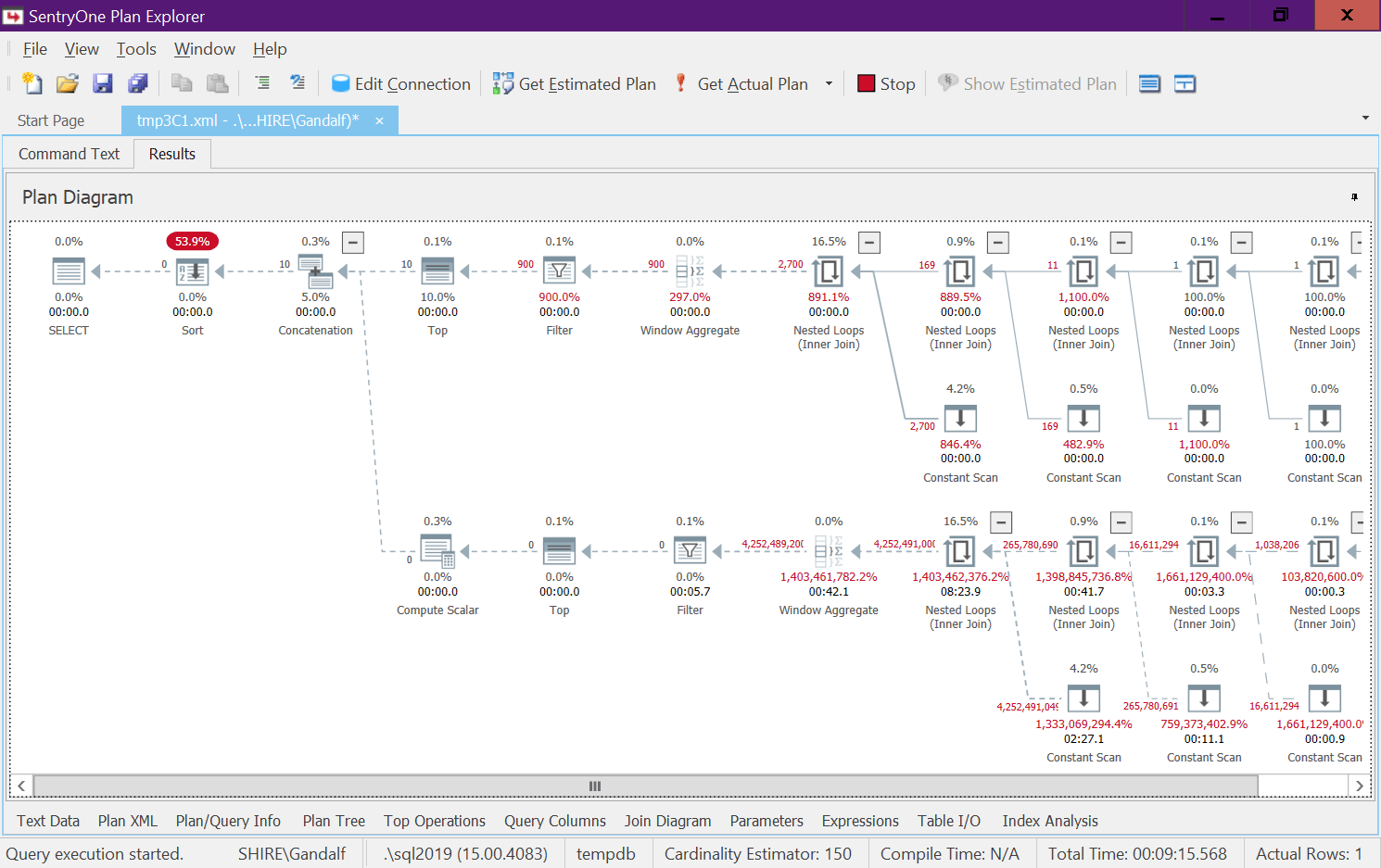Figure 8: Live Query Statistics for dbo.GetNumsAlanCharlieJoeItzikBatch(@mylow, @myhigh)

It took this test 9:15 minutes to complete on my machine, and remember that the request was to return a range of 10 numbers.

Let’s think if there’s a way to avoid activating the irrelevant subtree in its entirety. To achieve this, you would want the startup Filter operators to appear above the Top operators instead of below them. If you read Fundamentals of table expressions, Part 4 – Derived tables, optimization considerations, continued, you know that a TOP filter prevents unnesting of table expressions. So, all you need to do, is place the TOP query in a derived table, and apply the filter in an outer query against the derived table.

Here’s our modified function implementing this trick:

```CREATE OR ALTER FUNCTION dbo.GetNumsAlanCharlieJoeItzikBatch(@low AS BIGINT = 1, @high AS BIGINT)
RETURNS TABLE
AS
RETURN
WITH
L0 AS ( SELECT 1 AS c
FROM (VALUES(1),(1),(1),(1),(1),(1),(1),(1),
(1),(1),(1),(1),(1),(1),(1),(1)) AS D(c) ),
L1 AS ( SELECT 1 AS c FROM L0 AS A CROSS JOIN L0 AS B ),
L2 AS ( SELECT 1 AS c FROM L1 AS A CROSS JOIN L1 AS B ),
L3 AS ( SELECT 1 AS c FROM L2 AS A CROSS JOIN L2 AS B ),
Nums AS ( SELECT ROW_NUMBER() OVER(ORDER BY (SELECT NULL)) AS rownum
FROM L3 )
SELECT *
FROM ( SELECT TOP(@high)
rownum AS n,
@high + 1 - rownum AS op
FROM Nums LEFT OUTER JOIN dbo.BatchMe ON 1 = 0
ORDER BY rownum ) AS D1
WHERE @low = 1
UNION ALL
SELECT *
FROM ( SELECT TOP(@high - @low + 1)
@low - 1 + rownum AS n,
@high + 1 - rownum AS op
FROM Nums LEFT OUTER JOIN dbo.BatchMe ON 1 = 0
ORDER BY rownum ) AS D2
WHERE @low <> 1;
GO```

As expected, executions with constants keep behaving and performing the same as without the trick.

As for nonconstant inputs, now with small ranges it’s very fast. Here’s a test with a range of 10 numbers:

```DECLARE @mylow AS BIGINT = 1, @myhigh AS BIGINT = 10;

DECLARE @n AS BIGINT;

SELECT @n = n FROM dbo.GetNumsAlanCharlieJoeItzikBatch(@mylow, @myhigh) ORDER BY n OPTION(MAXDOP 1);```

The plan for this execution is shown in Figure 9.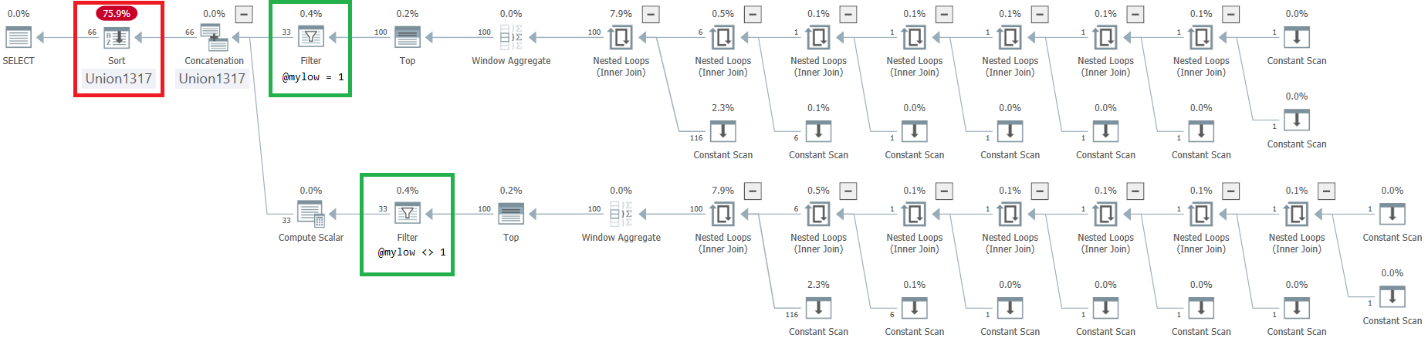Figure 9: Plan for improved dbo.GetNumsAlanCharlieJoeItzikBatch(@mylow, @myhigh)

Observe that the desired effect of placing the Filter operators above the Top operators was achieved. However, the ordering column n is treated as an outcome of manipulation, and therefore not considered an order preserving column with respect to rownum. Consequently, there’s explicit sorting in the plan.

Test the function with a large range of 100M numbers:

```DECLARE @mylow AS BIGINT = 1, @myhigh AS BIGINT = 100000000;

DECLARE @n AS BIGINT;

SELECT @n = n FROM dbo.GetNumsAlanCharlieJoeItzikBatch(@mylow, @myhigh) ORDER BY n OPTION(MAXDOP 1);```

I got the following time stats:

CPU Time = 29907 ms, elapsed time = 29909 ms.

What a bummer; it was almost perfect!

## Performance summary and insights

Figure 10 has a summary of the time statistics for the different solutions.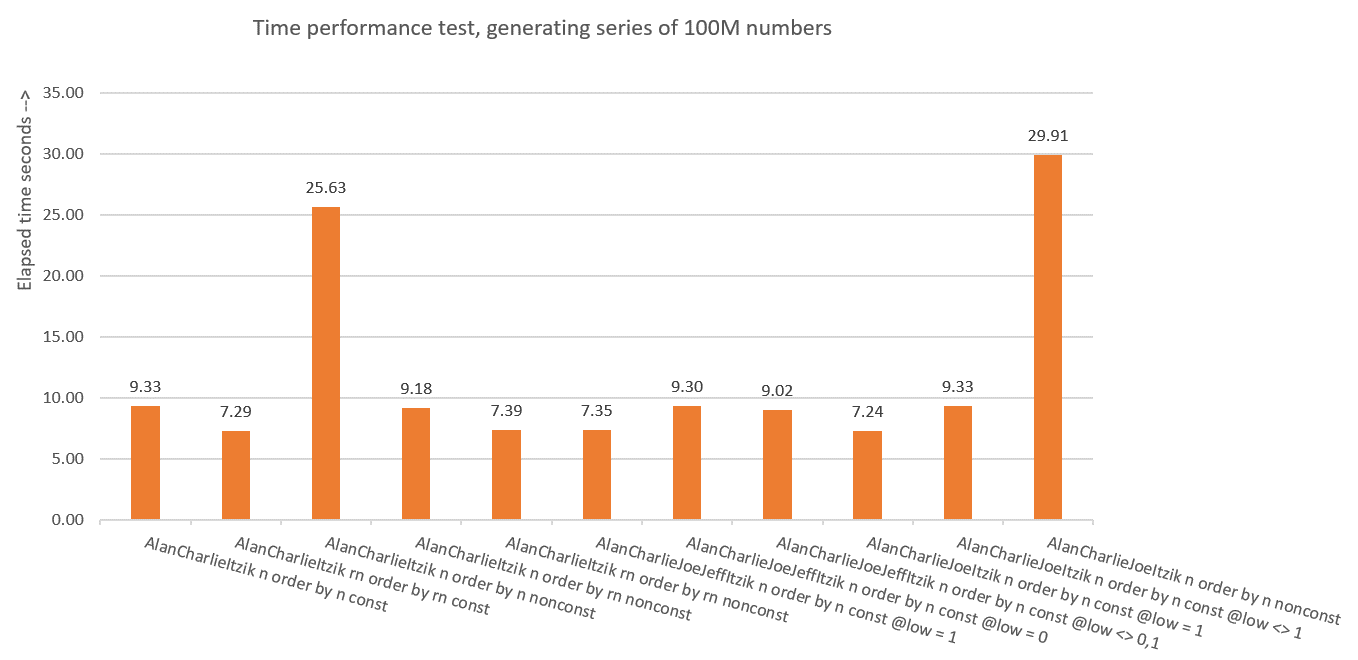Figure 10: Time performance summary of solutions

So what have we learned from all of this? I guess not to do it again! Just kidding. We learned that it’s safer to use the vertical elimination concept like in dbo.GetNumsAlanCharlieItzikBatch, which exposes both the nonmanipulated ROW_NUMBER result (rn) and the manipulated one (n). Just make sure that when needing to return the result ordered, always order by rn, whether returning rn or n.

If you’re absolutely certain that your solution will always be used with constants as inputs, you can use the horizontal unit elimination concept. This will result in a more intuitive solution for the user, since they will be interacting with one column for the ascending values. I’d still suggest using the trick with the derived tables to prevent unnesting and place the Filter operators above the Top operators if the function is ever used with nonconstant inputs, just to be on the safe side.

We’re still not done yet. Next month I’ll continue exploring additional solutions.